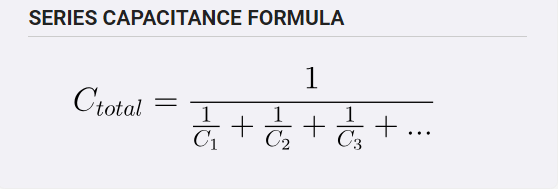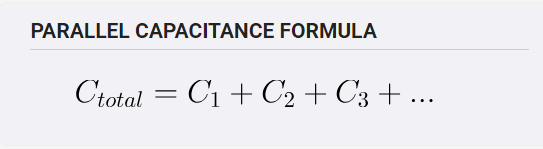# Calculating Capacitors in Parallel/Series

Here’s how to calculate the total amount of capacitance for Capacitors in Series and Parallel.

Let’s say we’re starting out with two capacitors, one 4 uF and one that is 6 uF.

Here is the formula for calculating their value if they’re in Series.Entering the example values into the equation yields:

C_{Total} = \dfrac{1} { \dfrac{1}{4\ uF } + \dfrac{1}{6\ uF}} = \dfrac{1} {0.25 + 0.167 } = 2.4\ uF

If they’re in parallel the formula would be:in which case 4uF + 6uF the answer would be 10uF.

If anyone is looking for assistance with calculating Parallel vs. Series capacitors DigiKey has a Handy Series/Parallel Capacitor Calculator located Here

>2 Likes